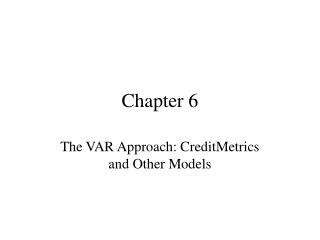# Chapter 6 - PowerPoint PPT PresentationDownload PresentationChapter 6

Chapter 6
Download Presentation## Chapter 6

- - - - - - - - - - - - - - - - - - - - - - - - - - - E N D - - - - - - - - - - - - - - - - - - - - - - - - - - -
##### Presentation Transcript

1. Chapter 6 The VAR Approach: CreditMetrics and Other Models

2. The Concept of VAR • Example of VAR applied to market risk. • Market price of equity = \$80 with estimated daily standard deviation = \$10. • If tomorrow is a “bad day” (1 in 100 worst) then what is the value at risk? • If normally distributed, then the cutoff is 2.33 below the mean = \$80 – 2.33(10) = \$56.70. 99% VAR = \$23.30 Figure 6.1.

3. CreditMetrics • What is VAR is next year is a “bad” year? • Since most loans are not publicly traded, then we do not observe the price or the standard deviation. • Consider VAR for an individual loan. Portfolios are covered in Chapter 11.

4. Rating Migration

5. Valuation • Consider BBB rated \$100 million face value loan with fixed 6% annual coupon and 5 years until maturity. Cash flow diagram – Figure 6.2.

6. Valuation at the End of the Credit Horizon (in 1 year) • Calculate one year forward zero yield curves plus credit spread (see Appendix 6.1)

7. Using the Forward Yield Curves to Value the Risky Loan • Under the credit event that the loan’s rating improves to A, the value at the end of yr 1: • Must repeat 8 times for each possible credit migration.

8. Table 6.3Value of the Loan at the End of Year 1 Under Different Ratings

9. Calculation of VARNormal vs. Actual DistributionFigure 6.3

10. Technical Problems • Rating Migration • Assumes stable Markov process. Nickell, Perraudin & Varotto (2001) find autocorrelation (2nd order process or higher) • Cyclical impact on transition matrix. Bangia, Diebold & Schuermann (2000). Figure 6.5 shows CreditMetrics Z macro shift factor: Finger (1999), Kim (1999). • Impact of bond “aging” on transition probabilities: Altman & Kishore (1997). Non-homogeneity within ratings classes: Kealhofer, Kwok, & Weng (1998). • Bond transition matrices not applicable to loans.

11. Technical Problems (cont.) • Valuation • Non-stochastic interest rates and credit spreads. • Fixed LGD. Volatility of LGD adds to VAR. • MTM vs. DM Models • VAR is less under DM than for MTM because DM does not consider loss of upside gain potential (if credit upgrades).

12. Appendix 6.1Calculating the Forward Zero Yield Curve for Valuation • Three steps: • Decompose current spot yield curve on risk-free (US Treasury) coupon bearing instruments into zero coupon spot risk-free yield curve. • Calculate one year forward risk-free yield curve. • Add on fixed credit spreads for each maturity and for each credit rating.

13. Step 1: Calculation of the Spot Zero Coupon Risk-free Yield Curve Using a No Arbitrage Method • Figure 6.6 shows spot yield curve for coupon bearing US Treasury securities. • Assuming par value coupon securities: • Figure 6.7 shows the zero coupon spot yield curve.

14. Step 2: Calculating the Forward Yields • Use the expectations hypothesis to calculate 6 month maturity forward yields:

15. Use the 6 month maturity forward yields to calculate the 1 year forward risk-free yield curve Figure 6.8

16. Step 3: Add on Credit Spreads to Obtain the Risky 1 Year Forward Zero Yield Curve • Add on credit spreads (eg., from Bridge Information Systems) to obtain FYCR in Figure 6.8.

17. Appendix 6.2Estimating Unexpected Losses Using Extreme Value Theory (EVT) • VAR measures the minimum loss that will occur with a certain probability. • EVT examines the size of the loss that exceeds VAR – the tail distribution. • Figure 6.4: Loss distribution is normal up until the 95%tile, shown to be a loss of \$4.93 million. After this, the distribution has the fat tails of a Generalized Pareto Distribution (GPD). • If used normal distribution then 99% VAR would be \$6.97 million. But under GPD, the 99% VAR is \$22.23 million.

18. Calculating the EVT VAR and the Expected Shortfall (ES) • The GPD is a 2 parameter skewed distribution: • With 10,000 observations, the 95% threshold is set by worst 500 observations.

19. Expected Shortfall = Mean of the excess distribution of unexpected losses beyond the threshold VAR • McNeil (1999) shows that: • Thus: 2.4 times more capital would be needed to secure the bank against catastrophic credit losses compared to unexpected losses occurring up to the 99th percentile level, even using the “fat tails” VAR measure. This may be excessive: Cruz, Coleman & Salkin (1998).